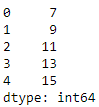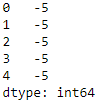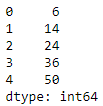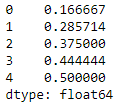# Add, subtract, multiple and divide two Pandas Series

• Last Updated : 28 Jul, 2020

Let us see how to perform basic arithmetic operations like addition, subtraction, multiplication, and division on 2 Pandas Series.

For all the 4 operations we will follow the basic algorithm :

1. Import the `Pandas `module.
2. Create 2 Pandas Series objects.
3. Perform the required arithmetic operation using the respective arithmetic operator between the 2 Series and assign the result to another Series.
4. Display the resultant Series.

 `# importing the module``import` `pandas as pd`` ` `# creating 2 Pandas Series``series1 ``=` `pd.Series([``1``, ``2``, ``3``, ``4``, ``5``])``series2 ``=` `pd.Series([``6``, ``7``, ``8``, ``9``, ``10``])`` ` `# adding the 2 Series``series3 ``=` `series1 ``+` `series2`` ` `# displaying the result``print``(series3)`

Output :### Subtraction of 2 Series

 `# importing the module``import` `pandas as pd`` ` `# creating 2 Pandas Series``series1 ``=` `pd.Series([``1``, ``2``, ``3``, ``4``, ``5``])``series2 ``=` `pd.Series([``6``, ``7``, ``8``, ``9``, ``10``])`` ` `# subtracting the 2 Series``series3 ``=` `series1 ``-` `series2`` ` `# displaying the result``print``(series3)`

Output :### Multiplication of 2 Series

 `# importing the module``import` `pandas as pd`` ` `# creating 2 Pandas Series``series1 ``=` `pd.Series([``1``, ``2``, ``3``, ``4``, ``5``])``series2 ``=` `pd.Series([``6``, ``7``, ``8``, ``9``, ``10``])`` ` `# multiplying the 2 Series``series3 ``=` `series1 ``*` `series2`` ` `# displaying the result``print``(series3)`

Output :### Division of 2 Series

 `# importing the module``import` `pandas as pd`` ` `# creating 2 Pandas Series``series1 ``=` `pd.Series([``1``, ``2``, ``3``, ``4``, ``5``])``series2 ``=` `pd.Series([``6``, ``7``, ``8``, ``9``, ``10``])`` ` `# dividing the 2 Series``series3 ``=` `series1 ``/` `series2`` ` `# displaying the result``print``(series3)`

Output :My Personal Notes arrow_drop_up